# 模拟大功率激光系统中的热致焦移

2014年 11月 18日

### 热透镜的原理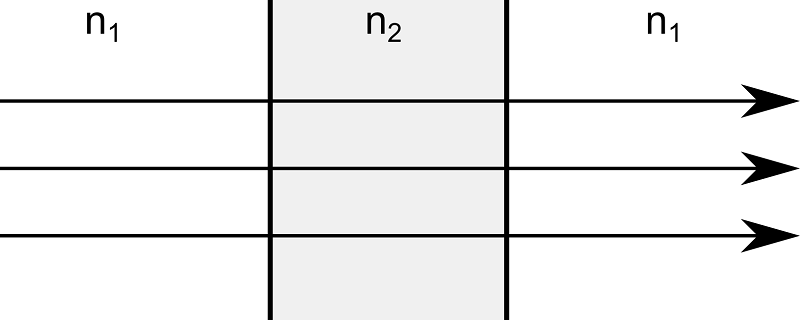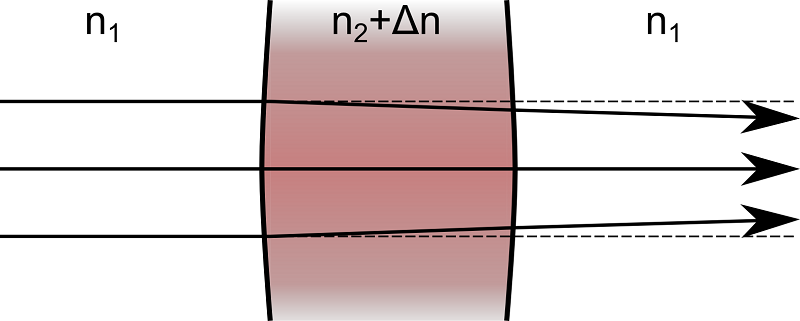### 模拟激光聚焦系统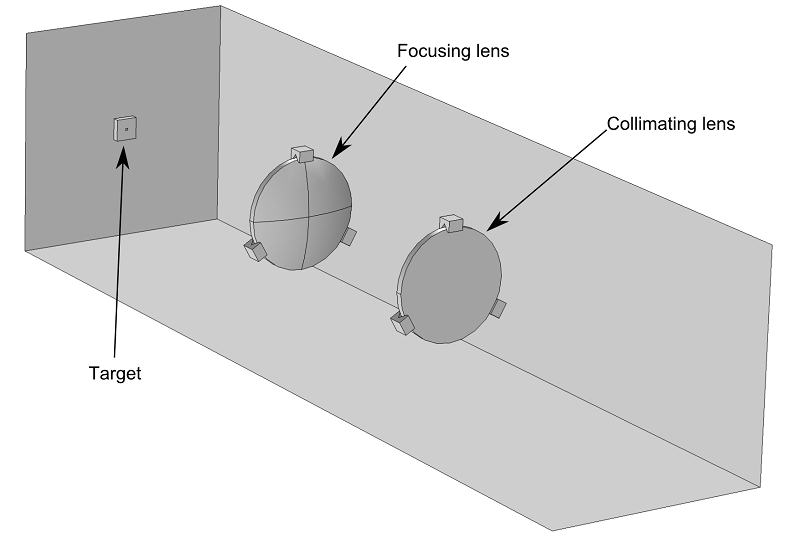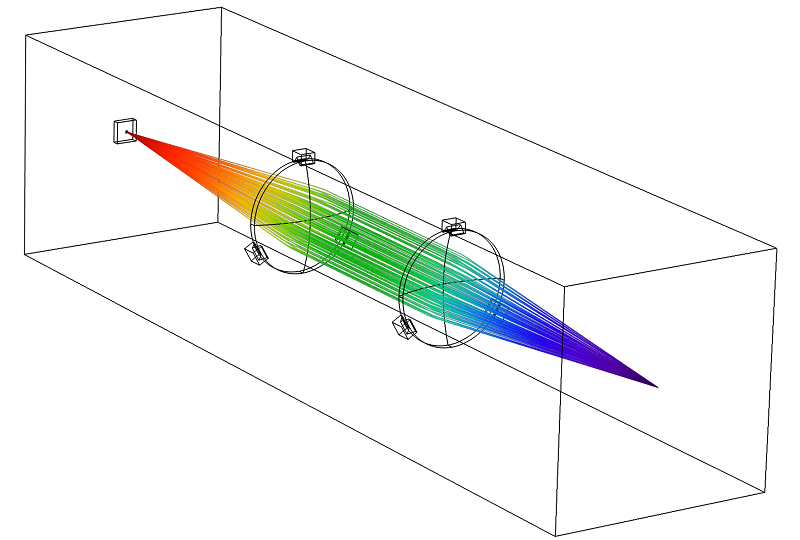### 吸收介质中的射线传播建模

• 几何光学 — 计算射线轨迹。
• 固体传热 — 计算透镜中的温度。
• 固体力学 — 模拟透镜的热膨胀。
• 动网格 — 使与透镜相邻的域中的有限元网格变形。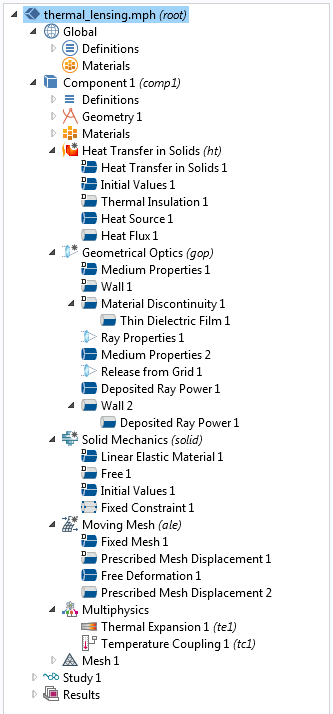#### 射线轨迹计算

(1)

\begin
{aligned}
\frac{d\mathbf{q}}{dt} &= \frac{\partial \omega}{\partial \mathbf{k}}\\
\frac{d\mathbf{k}}{dt} &= -\frac{\partial \omega}{\partial \mathbf{q}}
\end{aligned}

(2)

\omega = \frac{c\left|\mathbf{k}\right|}{n}

(3)

n_1 \sin(\theta_1) = n_2 \sin(\theta_2)

#### 计算每条射线传输的功率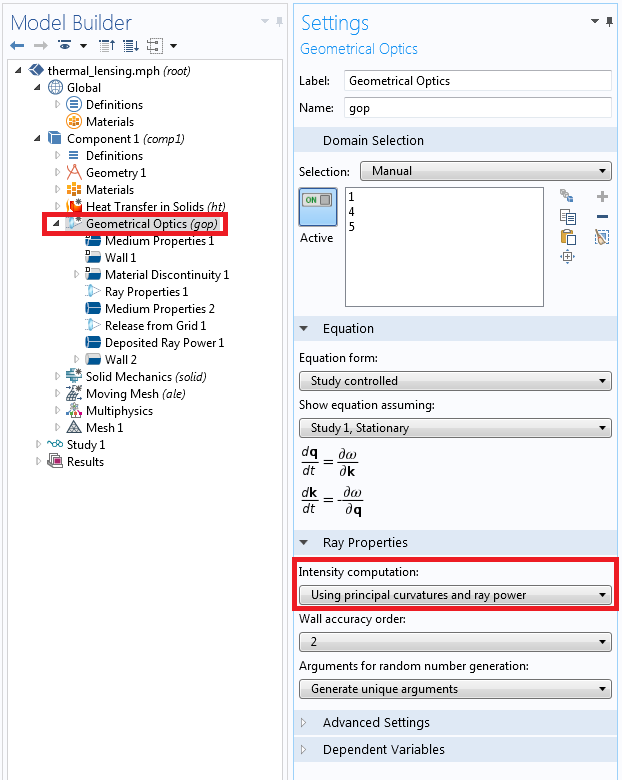(4)

Q(\mathbf{r})=Q(\mathbf{r}_0)\exp\left(-2k_0 \kappa\left|\mathbf{r}-\mathbf{r}_0\right|\right)

(5)

\frac{dQ_{\textrm{src}}}{dt} = -\sum_{j=1}^{N_t} \frac{dQ_j}{dt}\delta(\mathbf{r}-\mathbf{q}_j)

#### 计算温度

(6)

\nabla \cdot \left(-k\nabla T\right) = Q_{\textrm{src}}

(7)

-\mathbf{n}\cdot\left(-k\nabla T\right) = h\left(T_{\textrm{ext}}-T\right)

#### 结构变形

(8)

\epsilon_{\textrm{th}}=\alpha\left(T-T_{\textrm{ref}}\right)

### 获得合理的解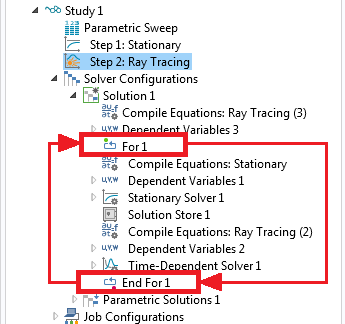For 和 End For 节点之间的节点将重复 For 节点的设置窗口中指定的次数。 此外，每次运行求解器时，它都会使用前一个求解器的解。 通过这种方式，可以在两个研究之间建立双向耦合，并在它们之间进行迭代，直到获得合理的解。

### 热透镜模拟结果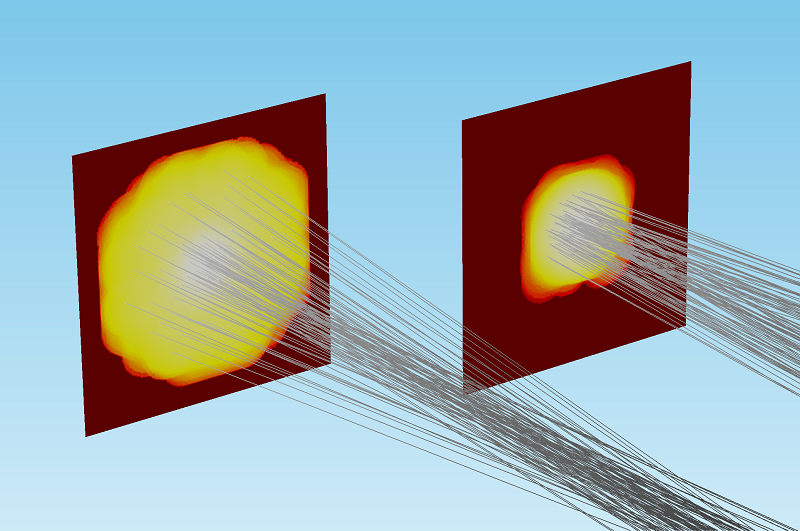3000w 光束（左）和 1w 光束（右）沉积射线功率的比较。 为了进行比较和可视化，沉积功率的颜色表达式已被归一化并用对数尺度表示。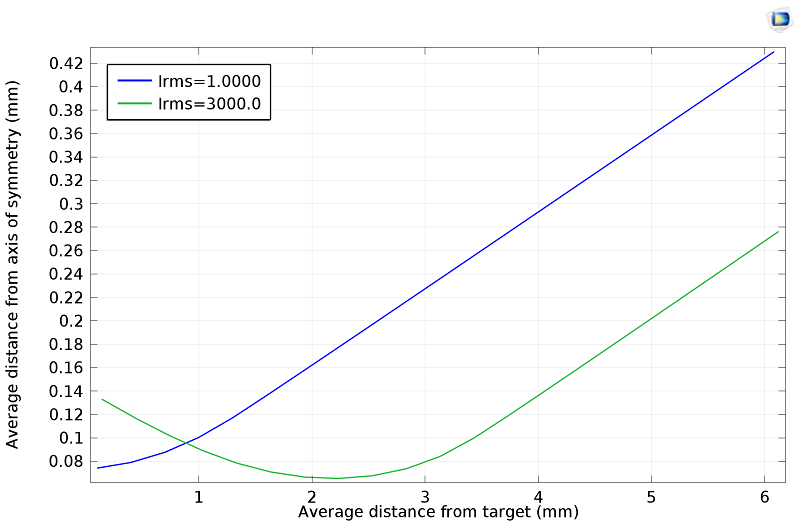### 模型下载

#### 评论 (2)

##### 留言##### 晨 苏
2023-09-17##### Min Yuan
2023-09-20 COMSOL 员工